Simplify Rational Expressions

Simplify Rational Expressions

Goal: Simplify Rational Expressions

Example

Simplify: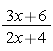Factor the numerator: 3x + 6 = 3(x + 2)

Factor the denominator: 2x + 4 = 2(x + 2)
Rewrite the expression in terms of the factors: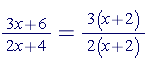The common factors in the numerator and denominator cancel out because any expression divided by itself is 1; therefore,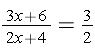Example

Simplify: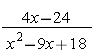Factor the numerator: x - 24 = 4(x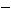6)

Factor the denominator: x2 - 9x + 18 = (x6)(x3)
Rewrite the expression in terms of the factors:=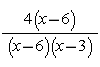Identify the common factors in the numerator and the denominator. These two factors cancel out because any expression divided by itself is 1.=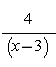It is important to know that rational expressions have restrictions on the variables so that denominators cannot equal zero. Thus, in the previous examples; x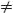2 and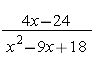;x3 or 6